# Numerical Determination of Eigenenergies for an Asymmetric Potential¶

## Examples - Quantum Mechanics¶

Last edited: October 31st 2018

In Numerical Determination of Eigeinenergies for the Harmonic Odcillator, we showed how to numerically determine the eigenenergies of the harmonic oscillator potential. Using the fact that the potential was symmetric, and hence the wave functions symmetric or anti-symmetric, we could easily choose the correct boundary conditions for the wave function we were interested in. However, for an asymmetric potential we don't have any such information! All we know is that the eigenfunctions have to be square integrable and smooth. And that is actually enough information to solve this problem.

Consider the following potential, $$V(x) = ax^4-b(x+c)^2+d,$$ with $a=1$, $b=1.5$, $c=0.2$, and $d=1.17$. The potential is slightly asymmetrical, as seen in the plot below.

In :
%matplotlib inline
from __future__ import division
import numpy as np
import matplotlib.pyplot as plt

# Set common figure parameters:
newparams = {'axes.labelsize': 14, 'axes.linewidth': 1, 'savefig.dpi': 300,
'lines.linewidth': 1.0, 'figure.figsize': (8, 3),
'figure.subplot.wspace': 0.4,
'ytick.labelsize': 10, 'xtick.labelsize': 10,
'legend.fontsize': 10, 'legend.frameon': False,
'legend.handlelength': 1.5}
plt.rcParams.update(newparams)

In :
n = 1000    # number of points per unit on x-axis
dx = 1.0/n
p = 10.0    # which x-values to include
linP = np.linspace(0,p*n-1, p*n, True)
linM = np.linspace(-(p*n-1), 0, p*n, True)
xP = linP/n # x-values in positive direction
xM = linM/n # x-values in negative direction

a = 1.0
b = 1.5
c = 0.2
d = 1.17
VP = a*xP**4 - b*(xP+c)**2 + d # potential for x>0
VM = a*xM**4 - b*(xM+c)**2 + d # potential for x<0

plt.figure()
plt.plot(xM, VM, 'r', xP, VP, 'r')
plt.grid()
plt.ylim([-1, 10])
plt.xlim([-2, 2])
plt.title(r'The Potential Under Consideration')
plt.xlabel(r'$x$')
plt.ylabel(r'$V(x)$');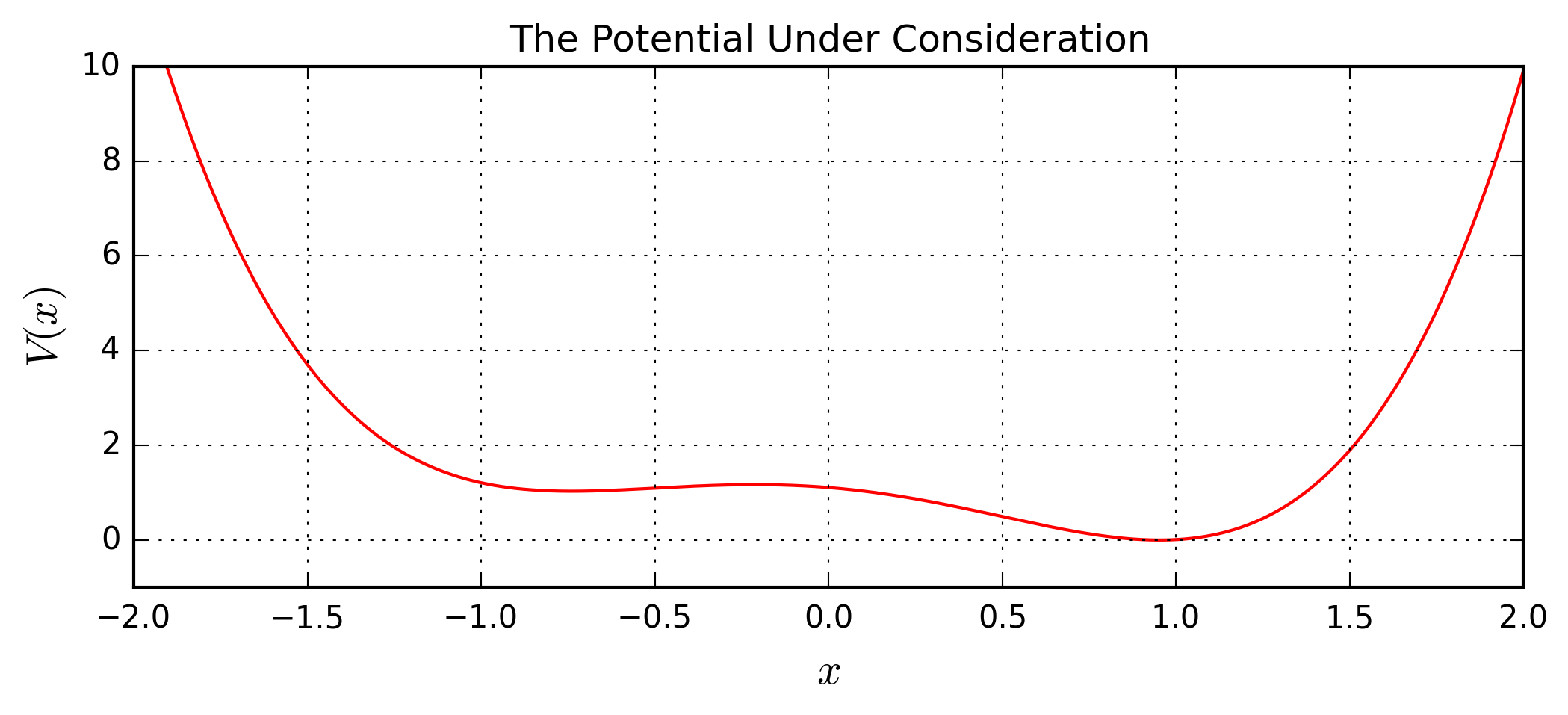Setting $\hbar = 1$, $m = 1$ for simplicity, the Schrödinger equation reads $$\left[-\frac{1}{2}\frac{\rm d^2}{{\rm d}x^2} + V(x) \right]\psi(x) = E \psi(x),$$ yielding the following equation for $\psi''(x)$, $$\psi''(x) = 2[V(x)-E]\psi(x).$$ Discretizing the $x$-axis and the wave function, and using the second-order central difference method, we get a formula for a function value $\psi_{i+1}$ based on two previous points, $$\psi_{i+1} = 2\psi_i-\psi_{i-1}-2(\Delta x)^2\left[E-V(x) \right]\psi_i,$$ where $\Delta x$ is the distance between two points $x_i$ and $x_{i+1}$ on the $x$-axis. For the first function value on each side of the origin we use $$\psi_1 = \psi_0 + \psi_0'\Delta x,$$ where $\psi_0$ and $\psi_0'$ are the initial value at the origin for the wave function and the slope of the wave function respectively.

As mentioned, we have to find functions that are square integrable and smooth. Hence we will try the following procedure: For a given energy, use the above equations to find wave functions for both positive and negative values of $x$ which are square integrable. To do this we need to define functions that find an initial value for the slope such that the wave functions go towards zero as $x \rightarrow \infty$, e.g. by using the bisection method. It is important to note that the criterion for the bisection method will vary depending on if the wave function approaches zero from above or below. Hence we need two different functions, and for simplicity we have also define seperate functions for positive and negative $x$-values. These four functions are defined below.

In :
def findSlopeP(Ein, acc):
"""Function for positive x, approaches zero from above.
Takes energy and desired accuracy as imput. """
S1 = -30.0    # lower limit for slope
S2 = 30.0     # upper limit for slope
DeltaSP = 1.0
S = 0         # starting value for slope
while DeltaSP > acc:
for i in linP[0:-1]:
if i == 0:
f1[i+1] = f1[i] + dx*S
else:
f1[i+1] = -f1[i-1] + f1[i]*(2-dx**2*2*(Ein-VP[i]))
if f1[i] < -20:
# the wave function shoots off towards minus infinity, adjust slope
S1 = S
S = S1 + (S2-S1)/2
break
elif f1[i] > 20:
# the wave function shoots off towards plus infinity, adjust slope
S2 = S
S = S2 - (S2-S1)/2
break
DeltaSP = S2-S1 # if DeltaSP is smaller than the given accuracy, the function returns the calculated slope
return S

def findSlopeM(Ein, acc):
"""Function for negative x, approaches zero from above. """
S1 = -30.0
S2 = 30.0
DeltaSM = 1.0
S = 0
while DeltaSM > acc:
for i in linP[1:-1]:
if i == 1:
f2[-(i+1)] = f2[-i] + dx*S
else:
f2[-(i+1)] = -f2[-(i-1)] + f2[-i]*(2-dx**2*2*(Ein-VM[-i]))
if f2[-i] <- 20:
S1 = S
S = S1 + (S2-S1)/2
break
elif f2[-i] > 20:
S2 = S
S = S2 - (S2-S1)/2
break
DeltaSM = (S2-S1)
return S

def findSlopeP2(Ein, acc):
"""Function for positive x, approaches zero from below. """
S1 = -30.0
S2 = 30.0
DeltaSP = 1.0
S = 0.0
while DeltaSP > acc:
for i in linP[0:-1]:
if i == 0:
f1[i+1] = f1[i] + dx*S
else:
f1[i+1] = -f1[i-1] + f1[i]*(2-dx**2*2*(Ein-VP[i]))
if f1[i] > 20:
S1 = S
S = S1 + (S2-S1)/2
break
elif f1[i] < -20:
S2 = S
S = S2 - (S2-S1)/2
break
DeltaSP = abs(S2-S1)
return S

def findSlopeM2(Ein, acc):
"""Function for negative x, approaches zero from below. """
S1 = -30.0
S2 = 30.0
DeltaSM = 1.0
S = 0.0
while DeltaSM > acc:
for i in linP[1:-1]:
if i == 1:
f2[-(i+1)] = f2[-i] + dx*S
else:
f2[-(i+1)] = -f2[-(i-1)] + f2[-i]*(2-dx**2*2*(Ein-VM[-i]))
if f2[-i] > 20:
S1 = S
S = S1 + (S2-S1)/2
break
elif f2[-i] < -20:
S2 = S
S = S2 - (S2-S1)/2
break
DeltaSM = abs(S2-S1)
return S


We now need to look for values of the energy which give the same slope in both directions. Using the fact that the $n^{\rm th}$ excited state has $n-1$ nodes, we can determine which combinations of the above functions to use. Now all is set to start computing the eigenenergies. We start with the ground state energy, $E_0$.

### Ground State¶

The ground state has zero nodes, hence we will use the functions where the wave function approaches zero from above, findSlopeP and findSlopeM, beginning the search in an interval $0<E<2$, with step length 0.1. Plotting the slope as a function of energy for both positive and negative values of $x$ will show if any of the energy values give a smooth wave function.

In :
f1 = np.zeros(p*n)
f1 = 1.0
f2 = np.zeros(p*n)
f2[-1] = 1.0

acc = 0.01
N = 2
E = np.linspace(0,N,10*N+1,True)
lin = np.linspace(0,10*N,10*N+1,True)
SP = np.zeros(10*N+1)
SM = np.zeros(10*N+1)
for k in lin:
SP[k] = findSlopeP(E[k], acc)
SM[k] = findSlopeM(E[k], acc)

plt.figure()
plt.plot(E, SP, 'b', E, -SM, 'g')
plt.xticks(np.arange(min(E), max(E), (max(E)-min(E))/10))
plt.grid()
plt.xlabel('Energy')
plt.ylabel('Slope');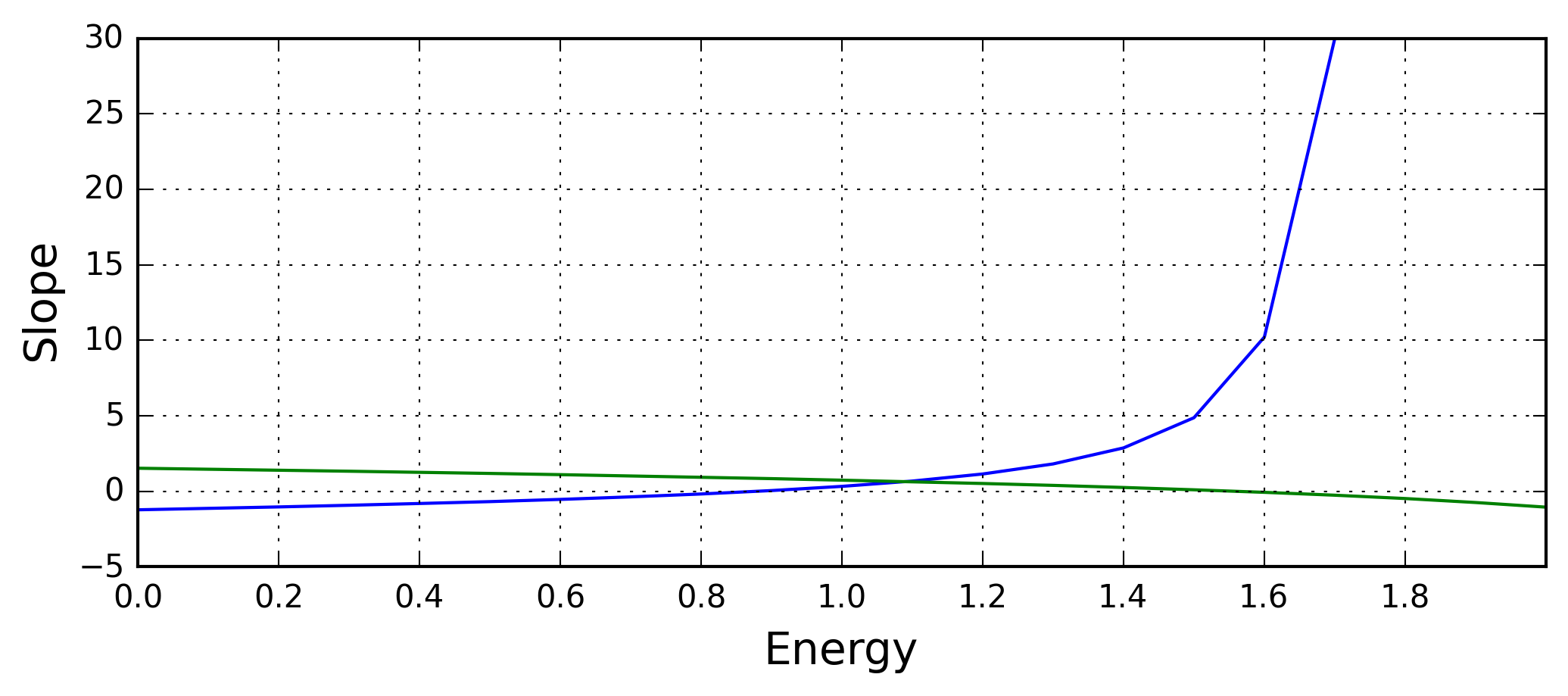We see that there is an intersection for an energy $E_0 \approx 1.1$. After some trial and error, we see that the ground state energy is approximately $E_0 = 1.09$ which gives a quite smooth function as seen below. So it seems like the method works!

In :
E0 = 1.09
f1 = np.zeros(p*n)
f1 = 1.0
f2 = np.zeros(p*n)
f2[-1] = 1.0
acc = 1e-6
SP0 = findSlopeP(E0, acc)
SM0 = findSlopeM(E0, acc)

print('Right slope: %s' % SP0)
print('Left slope: %s' % -SM0)

# Plot ground state
plt.figure()
plt.plot(xP, f1, 'b', xM, f2, 'b')
plt.xlim([-3,3])
plt.ylim([-0.5,2])
plt.ylabel('$\psi(x)$')
plt.xlabel('$x$')
plt.title('Ground State')
plt.grid();

Right slope: 0.635657161474
Left slope: 0.64026966691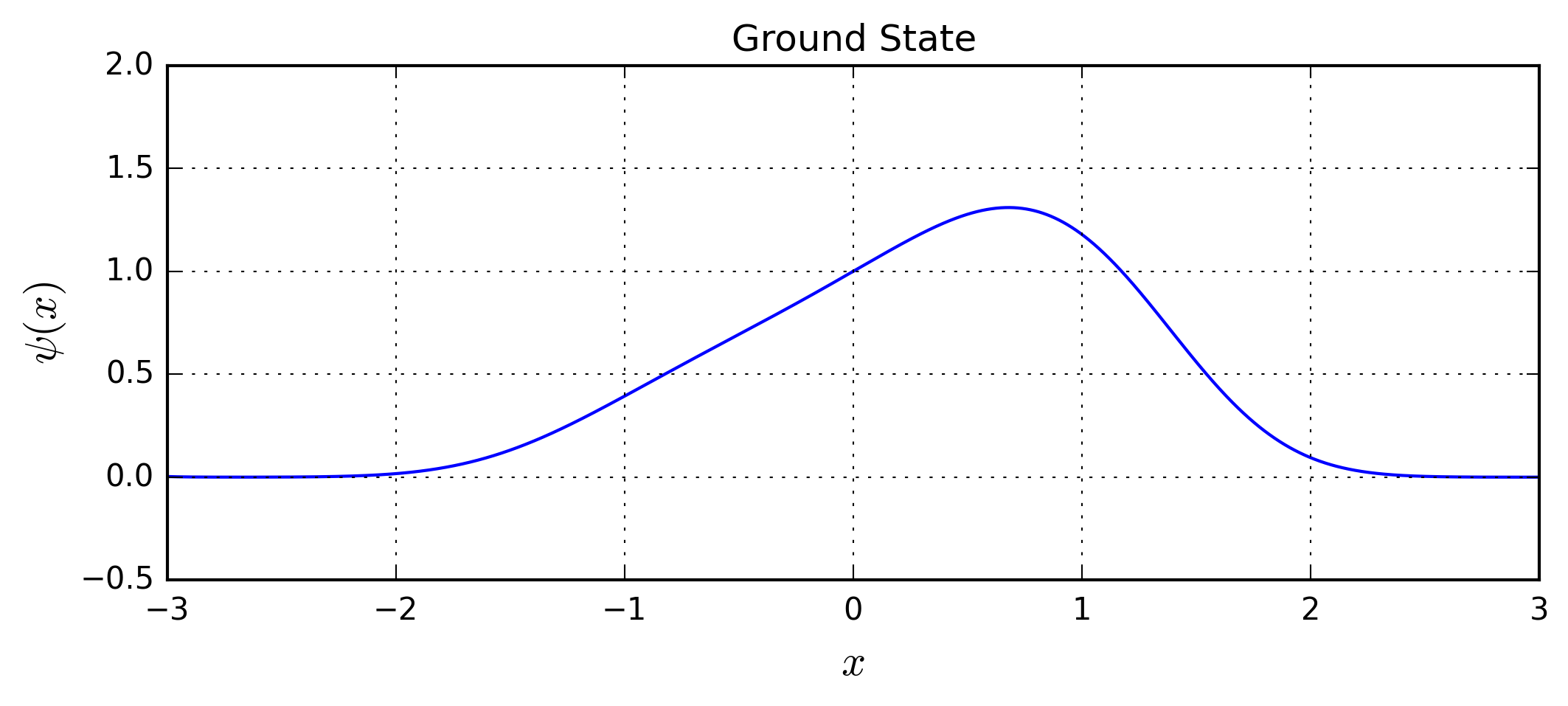We see now that the computed slopes are quite similar. To get higher accuracy we could have used a bisection method for energies close to $1.09$.

### First Excited State¶

For the first excited state, the node theorem states that we will have one node. Hence, the wave function will approach the $x$-axis from below on one side. But at which side of the origin will the node be? Looking at the plot of the potential above, we see that the potential is lower on the right side, and hence this area is more "allowed". Hence the node will probably lie to the right of the origin. To find $E_1$, we then have to use the second function, findSlopeP2, for positive values of $x$.

In :
f1 = np.zeros(p*n)
f1 = 1.0
f2 = np.zeros(p*n)
f2[-1] = 1.0

acc = 0.01
N = 2
start = 1
E = np.linspace(start, N+start, 10*N+1)
lin = np.linspace(0, 10*N, 10*N+1)
SP = np.zeros(10*N+1)
SM = np.zeros(10*N+1)
for k in lin:
SP[k] = findSlopeP2(E[k], acc)
SM[k] = findSlopeM(E[k], acc)

plt.figure()
plt.plot(E, SP, 'b', E, -SM, 'g')
plt.xticks(np.arange(min(E), max(E), (max(E)-min(E))/10))
plt.grid()
plt.xlabel('Energy')
plt.ylabel('Slope');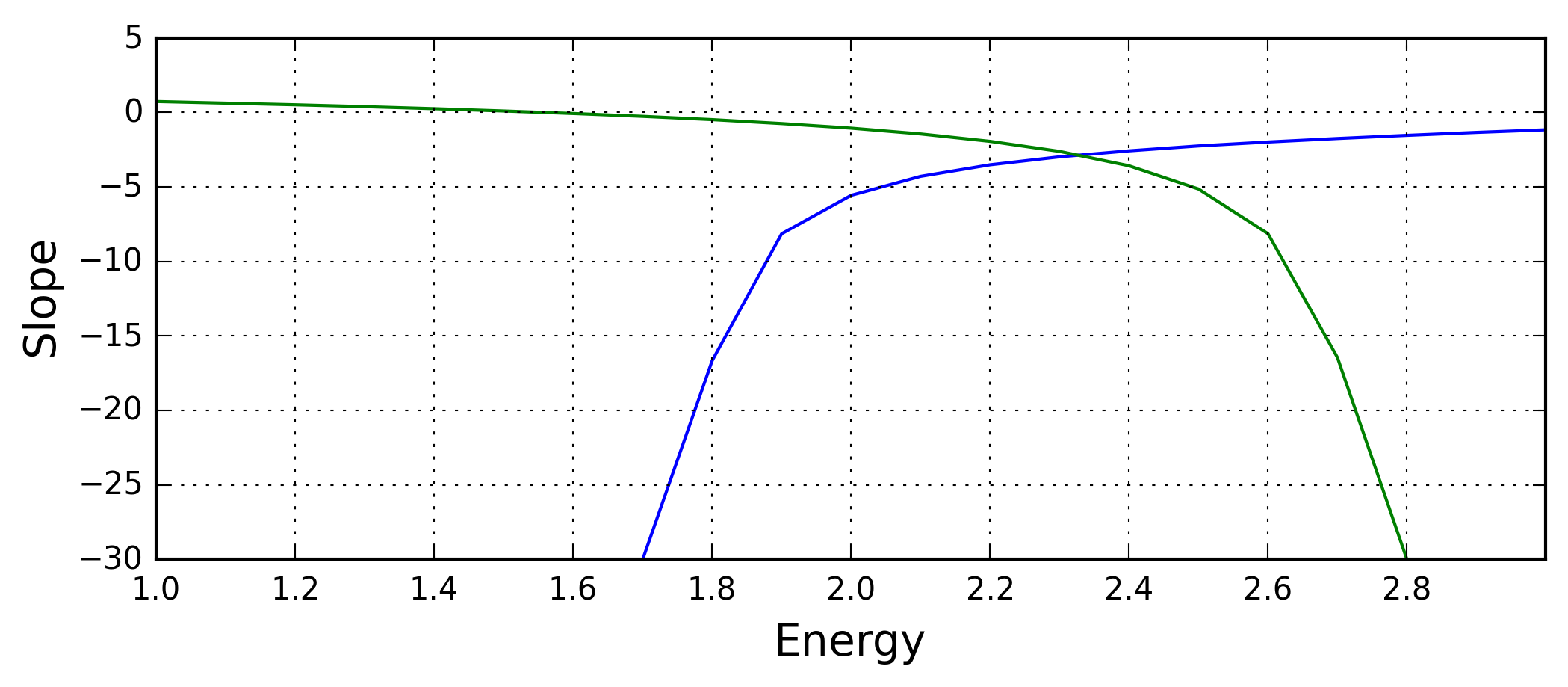Here we see that there is an intersection at $E_1 \approx 2.3$, or more accurately $E_1 = 2.33$.

In :
E1 = 2.33
f1 = np.zeros(p*n)
f1 = 1.0
f2 = np.zeros(p*n)
f2[-1] = 1.0
acc = 1e-6
SP1 = findSlopeP2(E1, acc)
SM1 = findSlopeM(E1, acc)

print('Right slope: %s' % SP1)
print('Left slope: %s' % -SM1)

# Plot first excited state
plt.figure()
plt.plot(xP, f1, 'b', xM, f2, 'b')
plt.xlim([-3,3])
plt.ylim([-2,2.5])
plt.ylabel(r'$\psi(x)$')
plt.xlabel(r'$x$')
plt.title('First Excited State')
plt.grid();

Right slope: -2.84302040935
Left slope: -2.85926297307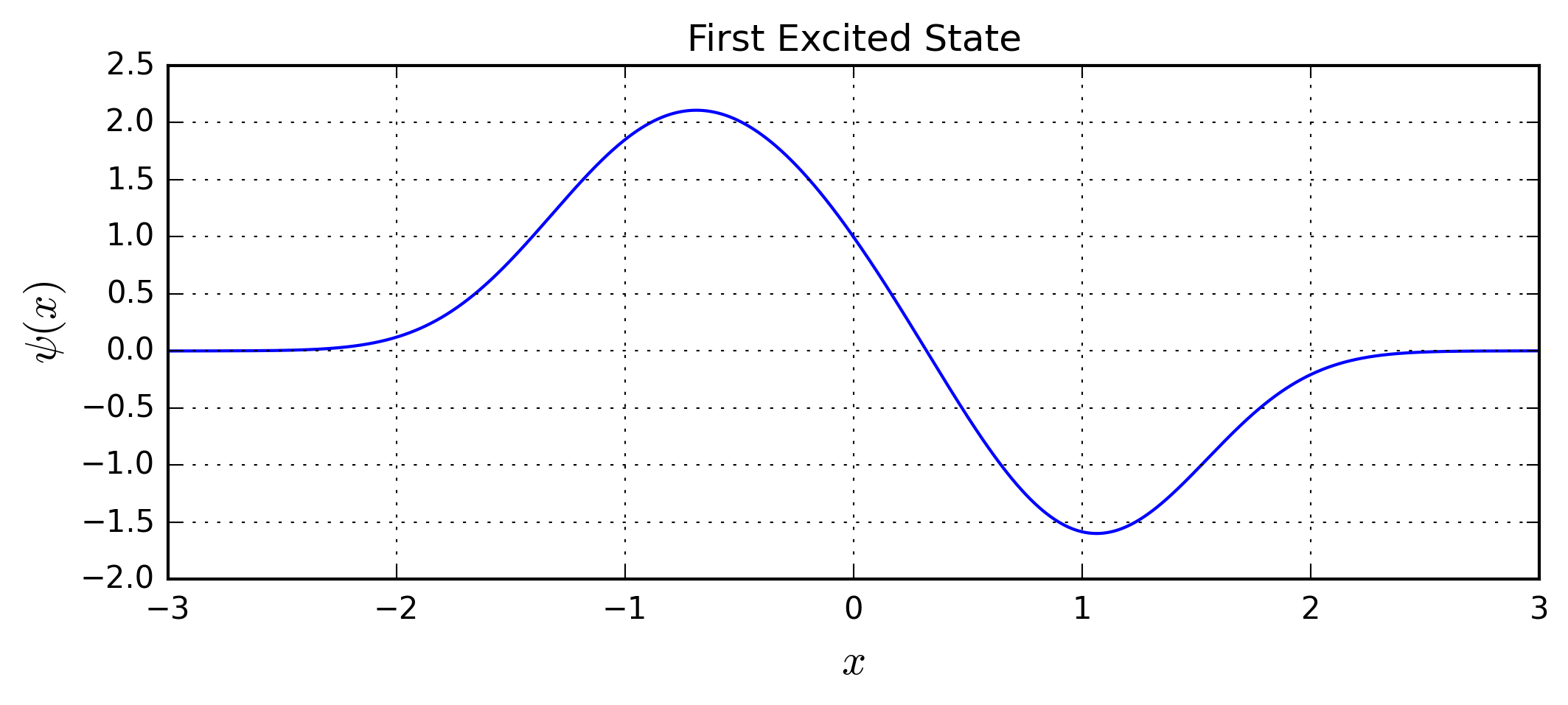### Second Excited State¶

For the second excited state, we expect two nodes, but where? Probably they will be on each side of the origin, but shifted to the right compared to a symmetric potential. Hence the wave function will approach the $x$-axis from below on both sides, making the second set of functions, findSlopeP2 and findSlopeM2, necessary for both negative and positive values of $x$.

In :
f1 = np.zeros(p*n)
f1 = 1.0
f2 = np.zeros(p*n)
f2[-1] = 1.0

acc = 0.01
N = 3
start = 2
E = np.linspace(start, N+start, 10*N+1)
lin = np.linspace(0, 10*N, 10*N+1)
SP = np.zeros(10*N+1)
SM = np.zeros(10*N+1)

for k in lin:
SP[k] = findSlopeP2(E[k], acc)
SM[k] = findSlopeM2(E[k], acc)

plt.figure()
plt.plot(E,(SP),'b',E,-(SM),'g')
plt.xticks(np.arange(min(E), max(E), (max(E)-min(E))/10))
plt.grid()
plt.xlabel('Energy')
plt.ylabel('Slope');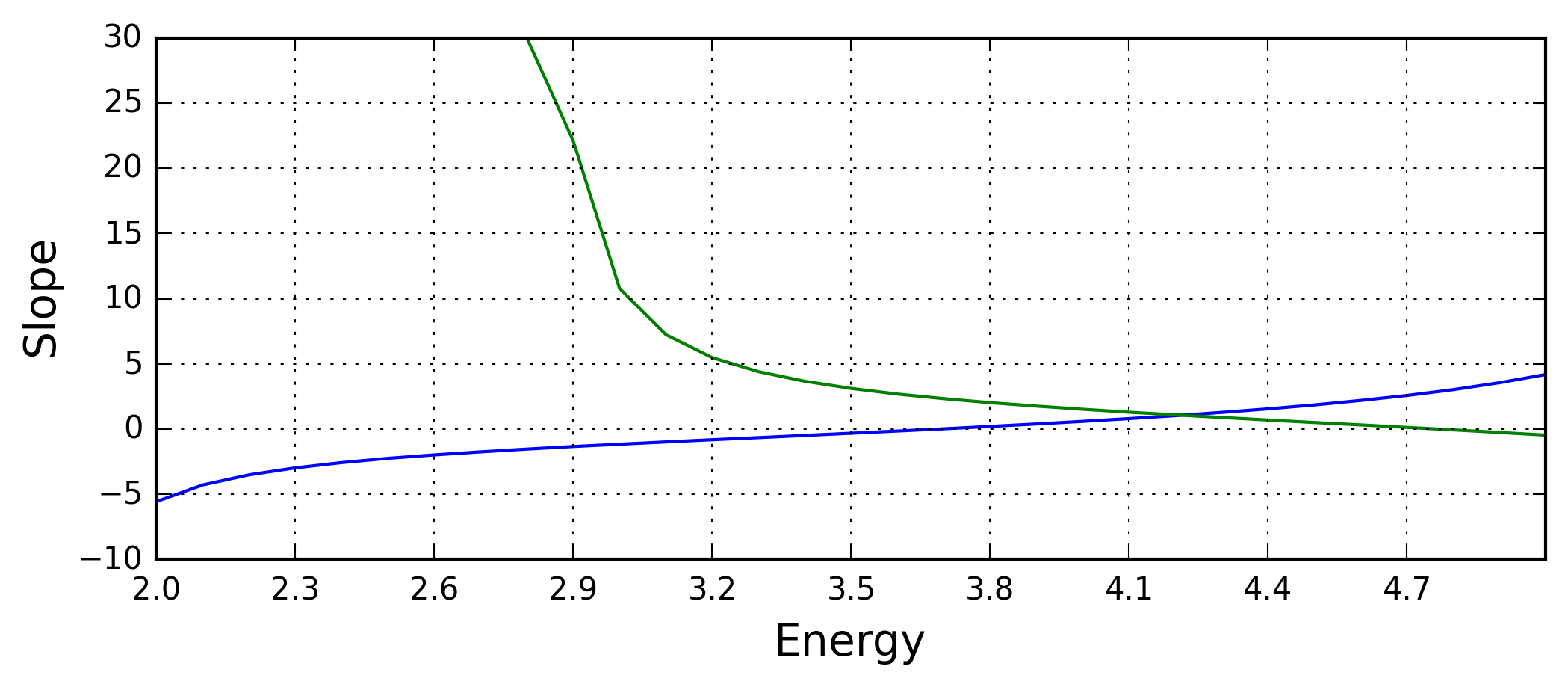The intersection is at $E_2 \approx 4.2$, and further investigation gives a more accurate value $E_2 = 4.21$.

In :
E2 = 4.21
f1 = np.zeros(p*n)
f1 = 1.0
f2 = np.zeros(p*n)
f2[-1] = 1.0
acc = 1e-6
SP2 = findSlopeP2(E2, acc)
SM2 = findSlopeM2(E2, acc)

print('Right slope: %s' % SP2)
print('Left slope: %s' % -SM2)

# Plot first excited state
plt.figure()
plt.plot(xP, f1, 'b', xM, f2, 'b')
plt.xlim([-3,3])
plt.ylim([-2,2.5])
plt.ylabel(r'$\psi(x)$')
plt.xlabel(r'$x$')
plt.title('Second Excited State')
plt.grid();

Right slope: 1.05427667499
Left slope: 1.06592908502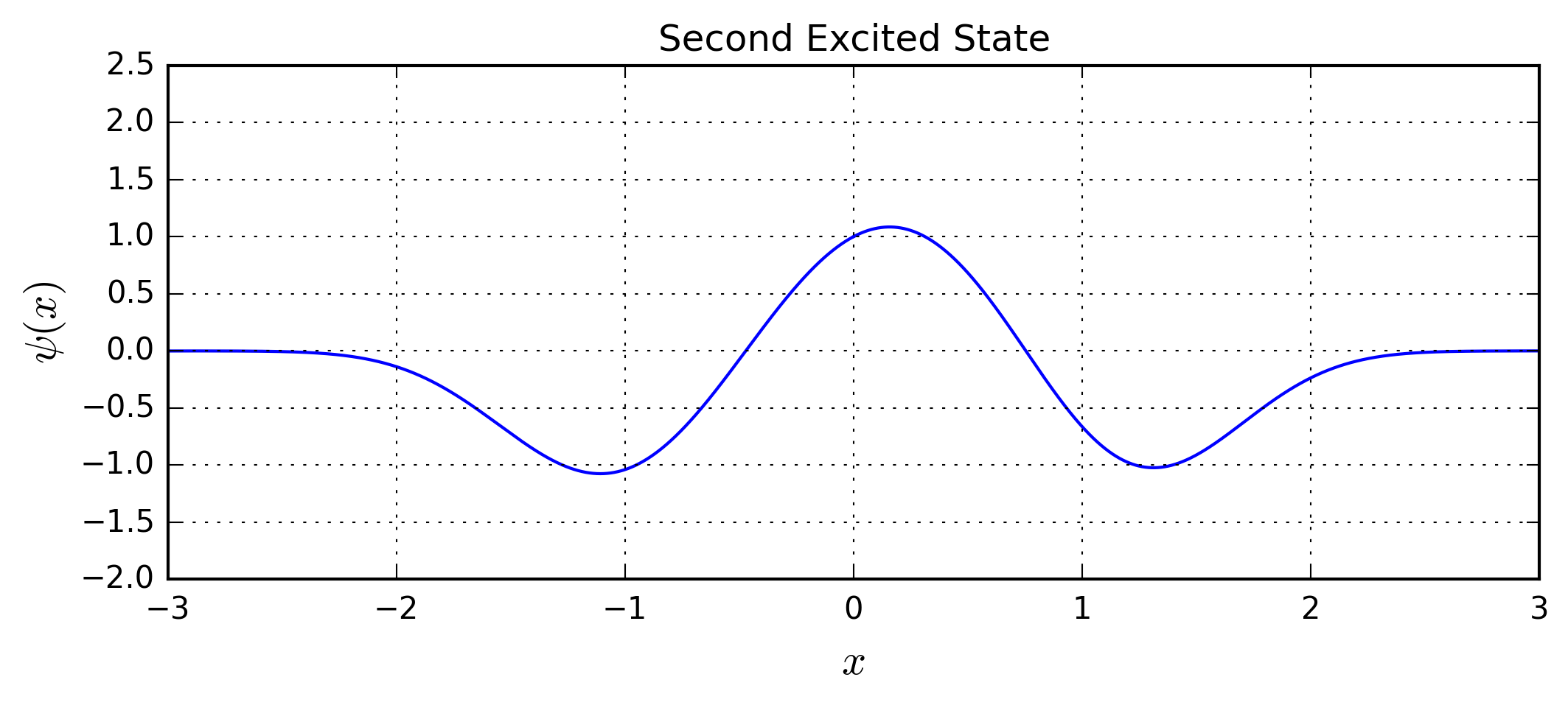We see from the plot above that the assumption regarding the nodes was correct. Continuing in a similar manner we could have determined the energies and plotted the wave functions for higher states as well. So, we have shown that one can in fact find the eigenenergies even for an asymmetric potential.